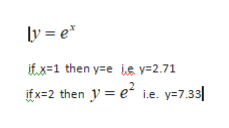# Can you explain me the linear growth and exponential growth and how to use it ?

Question
1 views

Can you explain me the linear growth and exponential growth and how to use it ?

check_circle

Step 1

linear growth can be easily explained by equation

Step 2

y=x

if  y=1 , then x=1

if y=2 , then x=2

implies when x is increasing then y is also increasing acco...help_outlineImage Transcriptioncloselye ifx 1 then yee y=2.71 ifx-2 then y e i.e. y=7.33| i.e. y7.33 fullscreen

### Want to see the full answer?

See Solution

#### Want to see this answer and more?

Solutions are written by subject experts who are available 24/7. Questions are typically answered within 1 hour.*

See Solution
*Response times may vary by subject and question.
Tagged in

### Algebra